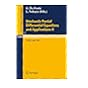Normal view

# Stochastic partial differential equations and applications : proceedings of a conference held in Trento, Italy, Sept. 30-Oct. 5, 1985 / edited by G. Da Prato and L. Tubaro.

Material type:TextSeries: Lecture notes in mathematics (Springer-Verlag) ; 1236.Publication details: ©1987. Description: 1 online resource (257 pages)Content type: text Media type: computer Carrier type: online resourceISBN: 9783540474081; 3540474080Additional physical formats: Print version:: Stochastic partial differential equations and applications.DDC classification: 519.2 LOC classification: QA3 | .L28 no. 1236 | QA274.25Other classification: SI 850 Online resources: Click here to access online
Contents:
Existence and uniqueness results for a non linear stochastic partial differential equation -- Continuity in non linear filtering some different approacees -- Expectation functionals associated with some stochastic evolution equations -- Dirichlet boundary value problem and optimal control for a stochastic distributed parameter system -- Stochastic product integration and stochastic equations -- Some remarks on a problem in stochastic optimal control -- Passage from two-parameters to infinite dimension -- The heat equation and fourier transforms of generalized brownian functionals -- The separation principle for stochastic differential equations with unbounded coefficients -- Weak convergence of measure valued processes using sobolev-imbedding techniques -- Probability distributions of solutions to some stochastic partial differential equations -- Two-sided stochastic calculus for spdes -- Convergence of implicit discretization schemes for linear differential equations with application to filtering -- Some applications of the Malliavin calculus to stochastic analysis -- Exit problem for infinite dimensional systems.
Action note: digitized 2010 committed to preserve
Tags from this library: No tags from this library for this title.
Star ratingsAverage rating: 0.0 (0 votes)
Holdings
Item type Current library Collection Call number Status Date due Barcode Item holdseBook e-Library

Electronic Book@IST

EBook Available
Total holds: 0

Includes bibliographical references.

Use copy Restrictions unspecified star MiAaHDL

Electronic reproduction. [Place of publication not identified] : HathiTrust Digital Library, 2010. MiAaHDL

Master and use copy. Digital master created according to Benchmark for Faithful Digital Reproductions of Monographs and Serials, Version 1. Digital Library Federation, December 2002. MiAaHDL

digitized 2010 HathiTrust Digital Library committed to preserve pda MiAaHDL

Print version record.

Existence and uniqueness results for a non linear stochastic partial differential equation -- Continuity in non linear filtering some different approacees -- Expectation functionals associated with some stochastic evolution equations -- Dirichlet boundary value problem and optimal control for a stochastic distributed parameter system -- Stochastic product integration and stochastic equations -- Some remarks on a problem in stochastic optimal control -- Passage from two-parameters to infinite dimension -- The heat equation and fourier transforms of generalized brownian functionals -- The separation principle for stochastic differential equations with unbounded coefficients -- Weak convergence of measure valued processes using sobolev-imbedding techniques -- Probability distributions of solutions to some stochastic partial differential equations -- Two-sided stochastic calculus for spdes -- Convergence of implicit discretization schemes for linear differential equations with application to filtering -- Some applications of the Malliavin calculus to stochastic analysis -- Exit problem for infinite dimensional systems.

## Other editions of this workStochastic partial differential equations and applications II : ©1989
Share